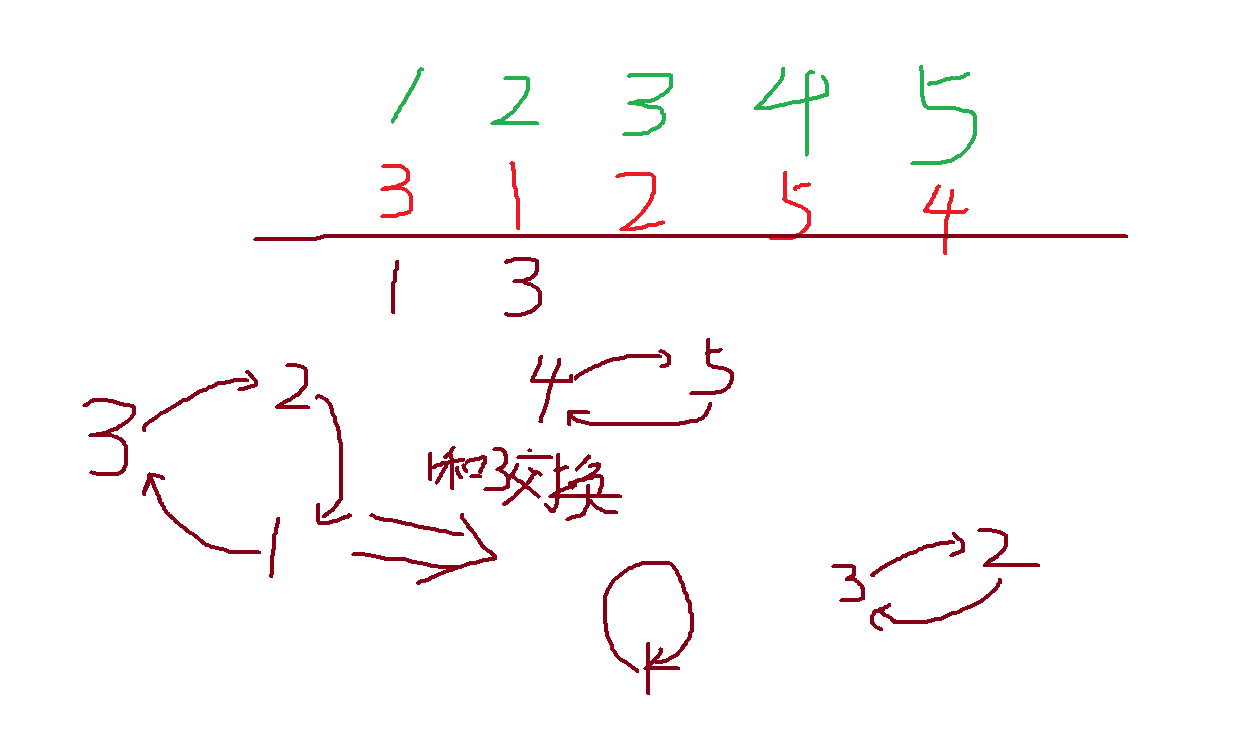# 双指针，BFS与图论（一）

2019/04/10 10:10

（一）双指针

1.日志统计

``````ts id
``````

1KN105,
0ts,id105,
1D10000

#### 输入样例：

``````7 10 2
0 1
0 10
10 10
10 1
9 1
100 3
100 3
``````

#### 输出样例：

``````1
3解题思路：排序+双指针①对所有的赞按时间排序②通过双指针i，j维护长度不大于d的区间，并记录该帖子中的获赞数``````
``````#include<iostream>
#include<algorithm>
#include<cstdio>
#define x first
#define y second
using namespace std;
typedef pair<int,int> PII;
const int N=100010;
int n,d,k;
int cnt[N];
bool ts[N];
PII flags[N];
int main()
{
int i,j;
scanf("%d%d%d",&n,&d,&k);
for(i=0;i<n;i++)
scanf("%d%d",&flags[i].x,&flags[i].y);
sort(flags,flags+n);
for(i=0,j=0;i<n;i++)
{
int id=flags[i].y;
cnt[id]++;

while(flags[i].x-flags[j].x>=d)
{
cnt[flags[j].y]--;
j++;
}
if(cnt[id]>=k)
ts[id]=true;
}
for(i=0;i<=100000;i++)
{
if(ts[i])
printf("%d\n",i);
}
return 0;
}``````

``（二）BFS1.献给阿尔吉侬的花束``

1<T10,
2R,C200

#### 输入样例：

``````3
3 4
.S..
###.
..E.
3 4
.S..
.E..
....
3 4
.S.. #### ..E. ``````

#### 输出样例：

``````5
1
oop!``````
``解题思路：要求最短的距离，应该用BFS来进行求解，我们需要map数组来存储地图，同时定义一个pair数组来存储当前或者下一步的位置，在定义一个vis数组来记录走的步数及是否走过该条路，每进行一次bfs都要对vis数组进行初始化。代码：``
``````#include<iostream>
#include<algorithm>
#include<queue>
#include<cstring>
#define x first
#define y second
using namespace std;
typedef pair<int,int> PII;
const int N=210;
int x[]={1,-1,0,0};
int y[]={0,0,1,-1};
int t,r,c;
char map[N][N];
int vis[N][N];

bool check(int X,int Y)
{
if(X<0||X>=r||Y<0||Y>=c)
return false;
if(map[X][Y]=='#')
return false;
if(vis[X][Y]!=0)
return false;
return true;
}
int bfs(int bx,int by)
{
queue<PII> q;
memset(vis,0,sizeof(vis));
vis[bx][by]=0;
PII m;
m.x=bx,m.y=by;
q.push(m);
while(q.size())
{
PII tem=q.front();
if(map[tem.x][tem.y]=='E')
return vis[tem.x][tem.y];
q.pop();
for(int i=0;i<4;i++)
{
int X=tem.x+x[i];
int Y=tem.y+y[i];
if(check(X,Y)==false)
continue;
vis[X][Y]=vis[tem.x][tem.y]+1;
PII tem2;
tem2.x=X,tem2.y=Y;
q.push(tem2);
}
}
return 0;
}
int main()
{
int i,j,bx,by;
cin>>t;
while(t--)
{
cin>>r>>c;
for(i=0;i<r;i++)
{
for(j=0;j<c;j++)
{
cin>>map[i][j];
if(map[i][j]=='S')
{
bx=i;
by=j;
}
}
}
int k=bfs(bx,by);
if(k)
{
cout<<k<<endl;
}
else
cout<<"oop!"<<endl;
}
return 0;
}``````

2.红与黑

#### 输入格式

1）‘.’：黑色的瓷砖；
2）‘#’：白色的瓷砖；
3）‘@’：黑色的瓷砖，并且你站在这块瓷砖上。该字符在每个数据集合中唯一出现一次。

1W,H20

#### 输入样例：

``````6 9
....#.
.....#
......
......
......
......
......
#@...#
.#..#.
0 0
``````

#### 输出样例：

``````45
``````
``解题思路：我们的目的是让交换的次数最少，看下图``

根据数字和对应的下标，将该数字与应该在的下标的数字相连，构成一个环，我们的目的是让每个数字自成一个环。

``````#include<iostream>
using namespace std;
const int N=10010;
int a[N];
bool tr[N];
int main()
{
int i,j,n;
int ans=0;
cin>>n;
for(i=1;i<=n;i++)
cin>>a[i];
for(i=1;i<=n;i++)
{
if(!tr[i])
{
ans++;
for(j=i;!tr[j];j=a[j])
tr[j]=true;
}
}
cout<<n-ans<<endl;
return 0;
}``````

`` ``

0
0 收藏

### 作者的其它热门文章0 评论
0 收藏
0